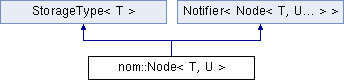Caffe2 - C++ API A deep learning, cross platform ML framework
nom::Node< T, U > Class Template Reference
Inheritance diagram for nom::Node< T, U >:Public Types

using NodeRef = typename Graph< T, U... >::NodeRef

using EdgeRef = typename Graph< T, U... >::EdgeRefPublic Types inherited from Notifier< Node< T, U... > >
using Callback = std::function< void(Node< T, U... > *)>

Public Member Functions

Node (T &&data)
Create a node with data.

Node ()
Create an empty node.

Node (Node &&)=default

Node (const Node &)=delete

Nodeoperator= (const Node &)=delete

Adds an edge by reference to known in-edges. More...

Adds an edge by reference to known out-edges. More...

void removeInEdge (EdgeRef e)
Removes an edge by reference to known in-edges. More...

void removeOutEdge (EdgeRef e)
Removes an edge by reference to known out-edges. More...

const std::vector< EdgeRef > & getOutEdges () const

const std::vector< EdgeRef > & getInEdges () const

void setInEdges (std::vector< EdgeRef > edges)

void setOutEdges (std::vector< EdgeRef > edges)Public Member Functions inherited from StorageType< T >
StorageType (T &&data)

StorageType (const T &data)=delete

const Tdata () const

TmutableData ()

void resetData (T &&data)Public Member Functions inherited from Notifier< Node< T, U... > >
Callback * registerDestructorCallback (Callback fn)

void deleteCallback (std::list< Callback > &callbackList, Callback *toDelete)

void deleteDestructorCallback (Callback *c)

void notify ()
Notifies all listeners (registerNotificationCallback users) of an update. More...

Friends

class Graph< T, U... >

Detailed Description

template<typename T, typename... U> class nom::Node< T, U >

Definition at line 32 of file Graph.h.

Member Function Documentation

template<typename T , typename... U>
 void nom::Node< T, U >::addInEdge ( EdgeRef e )
inline

Adds an edge by reference to known in-edges.

e A reference to an edge that will be added as an in-edge.

Definition at line 94 of file Graph.h.

template<typename T , typename... U>
 void nom::Node< T, U >::addOutEdge ( EdgeRef e )
inline

Adds an edge by reference to known out-edges.

e A reference to an edge that will be added as an out-edge.

Definition at line 100 of file Graph.h.

template<typename T , typename... U>
 void nom::Node< T, U >::removeInEdge ( EdgeRef e )
inline

Removes an edge by reference to known in-edges.

e A reference to an edge that will be removed from in-edges.

Definition at line 106 of file Graph.h.

template<typename T , typename... U>
 void nom::Node< T, U >::removeOutEdge ( EdgeRef e )
inline

Removes an edge by reference to known out-edges.

e A reference to an edge that will be removed from out-edges.

Definition at line 112 of file Graph.h.

The documentation for this class was generated from the following file:
• caffe2/core/nomnigraph/include/nomnigraph/Graph/Graph.h